GATE  >  Test: Power Systems- 2

# Test: Power Systems- 2

Test Description

## 20 Questions MCQ Test GATE Electrical Engineering (EE) 2023 Mock Test Series | Test: Power Systems- 2

Test: Power Systems- 2 for GATE 2023 is part of GATE Electrical Engineering (EE) 2023 Mock Test Series preparation. The Test: Power Systems- 2 questions and answers have been prepared according to the GATE exam syllabus.The Test: Power Systems- 2 MCQs are made for GATE 2023 Exam. Find important definitions, questions, notes, meanings, examples, exercises, MCQs and online tests for Test: Power Systems- 2 below.
Solutions of Test: Power Systems- 2 questions in English are available as part of our GATE Electrical Engineering (EE) 2023 Mock Test Series for GATE & Test: Power Systems- 2 solutions in Hindi for GATE Electrical Engineering (EE) 2023 Mock Test Series course. Download more important topics, notes, lectures and mock test series for GATE Exam by signing up for free. Attempt Test: Power Systems- 2 | 20 questions in 60 minutes | Mock test for GATE preparation | Free important questions MCQ to study GATE Electrical Engineering (EE) 2023 Mock Test Series for GATE Exam | Download free PDF with solutions
 1 Crore+ students have signed up on EduRev. Have you?
Test: Power Systems- 2 - Question 1

### Three generators rated 100 MVA, 11 kV have an impedance of 0.15pu each. If in the same plant, these generators are being replaced by a single equivalent generator, the effective impedance of equivalent generator will be

Detailed Solution for Test: Power Systems- 2 - Question 1

Applying Thevenin's theorm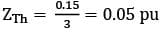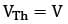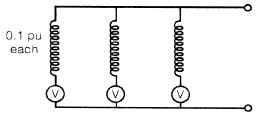Test: Power Systems- 2 - Question 2

### If all the sequence voltages at the fault point in a power system are equal, then the fault is a

Detailed Solution for Test: Power Systems- 2 - Question 2

For a solid fault i.e, Zf = 0, Va1 = Va2 = Va0 for L-L-G fault.

Test: Power Systems- 2 - Question 3

### A three-phase transformer having zero-sequence impedance of Z0 has the zero-sequence network as shown in the figure below. The connections of its windings are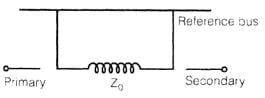Test: Power Systems- 2 - Question 4

Which one of the following relays has the capability of anticipating the possible major fault in a transformer?

Detailed Solution for Test: Power Systems- 2 - Question 4

Buchholz relay takes care of all incipient internal faults.

Test: Power Systems- 2 - Question 5

In a 220 kV system, the inductance and capacitance up to the circuit breaker location are 25 mH and 0.025 μF respectively. The value of resistor required to be connected across the breaker contacts which will give no transient oscillations, is

Detailed Solution for Test: Power Systems- 2 - Question 5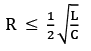or no transient oscillation.

Test: Power Systems- 2 - Question 6

The operating characteristic of a distance relay in the R-X plane is shown in the figure below, It represents operating characteristic of a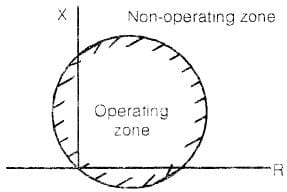Test: Power Systems- 2 - Question 7

Two power plants interconnected by a tie-line as shown in the below figure have loss formula coefficient B11 = 10-3 MW-1. Power is being dispatched economically with plant '1' as 100 MW and plant ꞌ2' as 125 MW. The penalty factors for plants 1 and 2 are respectively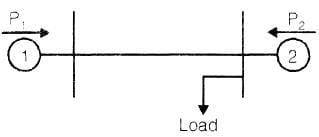Detailed Solution for Test: Power Systems- 2 - Question 7

Since the load is at the bus of plant-2, therefore, the line loss will not be affected by variation of P2 . Thus B12 = B21 = 0 and B22 = 0
For a 2-plant system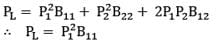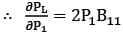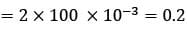∴ Penalty factor for plant-1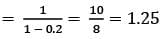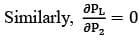∴ Penalty factor for plant-2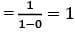Penalty factor is given by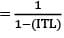Where ITL means incremental transmission loss.

Test: Power Systems- 2 - Question 8

The below diagram shows the layout of a power station having two generators A and B, connected to the 11 kV buses which are also fed through two transformers C and D from a 132 kV grid. The 11 kV buses are interconnected through a reactor R.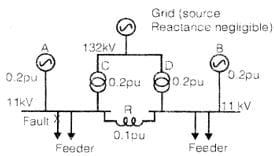The reactance of A, B, C, D and R in p.u. on a common MVA and kV-base. All the generated voltages in A, B and grid are each 1.0 p.u. and assumed as in phase at the time of fault.
The steady state symmetrical fault-current for a 3-phase fault on the 11 kV feeders is

Detailed Solution for Test: Power Systems- 2 - Question 8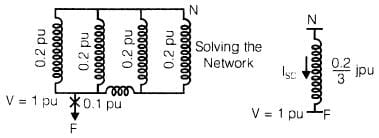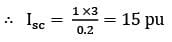Test: Power Systems- 2 - Question 9

The speed regulation parameter R of a control area is 0.025 Hz/MW and load frequency constant D is 2 MW/Hz, The area frequency response characteristic (AFRC) is

Detailed Solution for Test: Power Systems- 2 - Question 9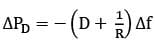∴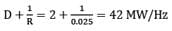Where, Δf → change in frequency and ΔPD → change in load demand.

Test: Power Systems- 2 - Question 10

The per-unit impedance of a circuit element of 0.15. If the base kV and base MVA are halved, then the new value of the per-unit impedance of the circuit element will be

Detailed Solution for Test: Power Systems- 2 - Question 10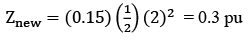Test: Power Systems- 2 - Question 11

The per-unit impedance of an alternator corresponding to base values 13.2 kV and 30 MVA is 0.2 p.u. The p.u. value of the impedance for base values 13.8kV and 50 MVA in p.u. will be

Detailed Solution for Test: Power Systems- 2 - Question 11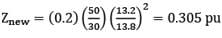Test: Power Systems- 2 - Question 12

For a given base voltage and base volt-amp, the per unit impedance value of an element is X. What will be the per-unit impedance value of this element when the voltage andvolt-amp bases are both doubled?

Detailed Solution for Test: Power Systems- 2 - Question 12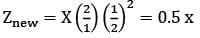Test: Power Systems- 2 - Question 13

The reflection coefficient for the transmission line shown in figure below at point P is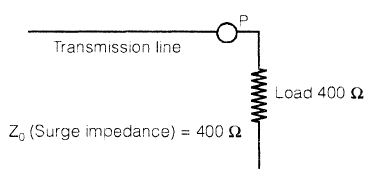Detailed Solution for Test: Power Systems- 2 - Question 13

The line is terminated into its surge impedance so it is an infinite line.

Test: Power Systems- 2 - Question 14

A 3-phase transmission line has its conductors at the corners of an equilateral triangle with side 3 m. The diameter of each conductor is 1.63 cm. The inductance of the line per phase per km is

Detailed Solution for Test: Power Systems- 2 - Question 14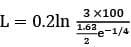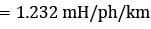Test: Power Systems- 2 - Question 15

A cable has inductance of 0.22 mH per km and capacitance of 0.202 μF per km. The surge impedance of the cable is

Detailed Solution for Test: Power Systems- 2 - Question 15

Z0 =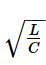Test: Power Systems- 2 - Question 16

For some given transmission line the expression for voltage regulation is given by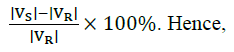Detailed Solution for Test: Power Systems- 2 - Question 16

In case of a short line, when load is removed, the voltage at the receiving end is equal to the voltage at the sending end. At full load |VR, Fl| = |VR| and at no load, |VR, nl| = |VS|

Test: Power Systems- 2 - Question 17

In a certain single-phase a.c. circuit the instantaneous voltage is given by v = V sin (ωt + 30°) p.u. and the instantaneous current is given by i = I sin (ωt - 30°) p.u. Hence the per unit value of reactive power is

Detailed Solution for Test: Power Systems- 2 - Question 17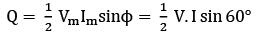Test: Power Systems- 2 - Question 18

A surge of l00 kV travels along an overhead line towards its junction with a cable. The surge impedance for the overhead line and cable are 400 ohms and 50 ohms respectively. The magnitude of the surge transmitted through the cable is

Detailed Solution for Test: Power Systems- 2 - Question 18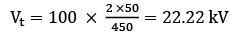Test: Power Systems- 2 - Question 19

A surge voltage rising at 100 kV/μs travels along a loss-less open-circuited transmission line. It takes 10 μs to reach the open end. The reflected wave from the open end, will be rising at

Detailed Solution for Test: Power Systems- 2 - Question 19

VR = Vi =  100 × 10 = 1000V/µs

As Z2 = ∞

Test: Power Systems- 2 - Question 20

At slack bus, which one of the following combinations of variables is not specified?

Detailed Solution for Test: Power Systems- 2 - Question 20

Load bus: Known quantities - P and Q Unknown quantities - V and δ

Generator bus: Known quantities - P and V Unknown quantities - Q and δ

Slack or reference bus: Known quantities - V and δ Unknown quantities - P and Q

## GATE Electrical Engineering (EE) 2023 Mock Test Series

22 docs|274 tests
Information about Test: Power Systems- 2 Page
In this test you can find the Exam questions for Test: Power Systems- 2 solved & explained in the simplest way possible. Besides giving Questions and answers for Test: Power Systems- 2, EduRev gives you an ample number of Online tests for practice

## GATE Electrical Engineering (EE) 2023 Mock Test Series

22 docs|274 tests(Scan QR code)